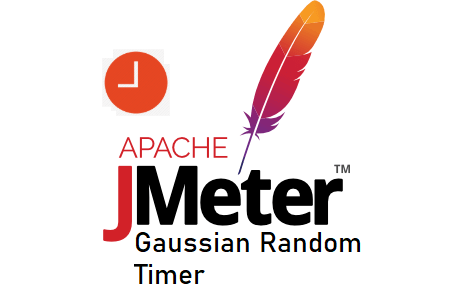# JMeter – Gaussian Random TimerAs the name suggested, JMeter Gaussian Random Timer is used to generate and add the random delay before the execution of a sampler. This timer is based on the Normal or Gaussian Distribution Function. The delay (think) time is the sum of the Gaussian distributed value (with mean 0.0 and standard deviation 1.0) times the deviation value you specify and the offset value.

## How to add ‘Gaussian Random Timer’?

Follow the below steps to add the Gaussian Random Timer in the script:

1. Select any appropriate element from the below list where you want to add the timer
1. Test Plan
3. Controller
4. Sampler
2. Right-click on the element
3. Hover the mouse on ‘Add’
4. Hover the mouse on ‘Timers’
5. Click ‘Gaussian Random Timer’

## What are the input fields ‘Gaussian Random Timer’?

‘Gaussian Random Timer’ has following input fields:

1. Name: To provide the name of the timer
3. Deviation (in milliseconds): The number shows how much the delay can deviate from the given offset towards the higher and lower range.
4. Constant Delay Offset (in milliseconds): The number shows a constant delay which will be added in random number generated by Gaussian Function in the range of given deviation value.

## Example:

If Constant Delay Offset is 1000 and deviation is 200 then Gaussian Random Timer will generate the random number in the range of 800 to 1200 milliseconds:

Gaussian Random Timer Delay = 1000 + GF*200

where GF value could be from -1 to 1